# Compound Interest

Updated January 13, 2021

The financial world often refers to compound interest as magic. Compound interest can be thought of as “interest building on interest” which adds to your principal. In layman’s terms, it’s a fundamental way to build wealth with little effort.

Compound interest isn’t as magical, however, when it occurs on a loan. In this case, it works for the lender – and against you.

## What Is Compound Interest?

Compound interest is defined as interest earned on principal plus interest that was earned previously.

Compound interest is important to understand if you:

• Invest money

• Save money in an account that earns interest

• Have debt and don’t pay off your interest each period

For example, when you deposit money into a high yield savings account and leave it there, that money will collect a certain amount of interest each month. The bank is paying you interest for keeping your money with them. That monthly interest is added to your principal (original deposit) and interest collects again the next month. It’s a cycle where interest earns interest and your money works for you while you sleep.

## How to Calculate Compound Interest

The formula for compounding interest is: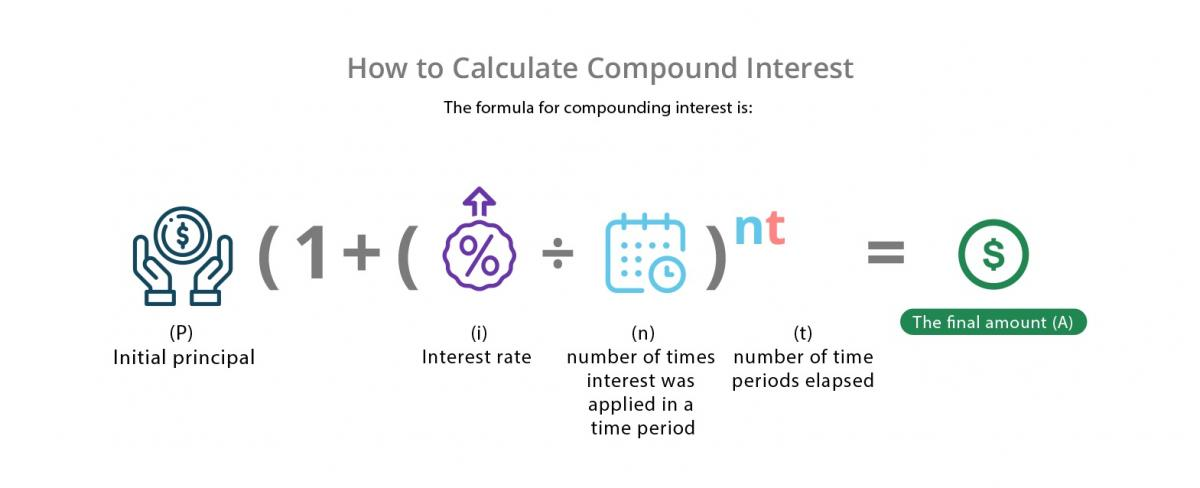If you don’t want to work out this formula, you can find a compound interest calculator that will do the calculation for you.

### How to Calculate Compound Interest in Excel

There are several ways to calculate compounding interest in Excel. A quick way is to use the following formula:

A=PV(1+i)n

A= The final amount

PV= Current principal value

i=interest rate

n= number of compounding periods (frequency)

#### Compound Interest in Excel Example

Let’s say you have \$10,000 and an interest rate of 5%. The interest is collected every month, so it compounds monthly. In three years, your initial investment of \$10,000 will have grown to \$11,615 (if you don’t touch it).

Here’s an example of how that calculation looks in Excel: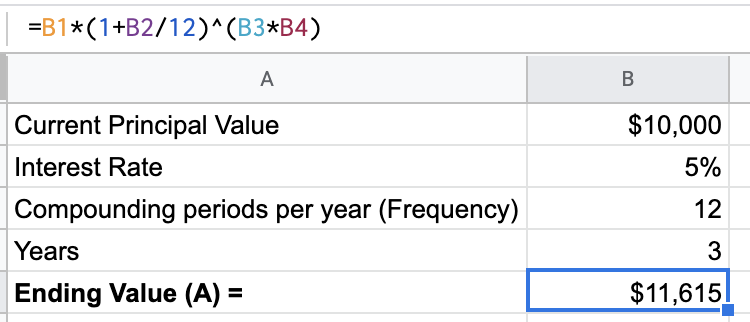The excel formula rounds up. Running these same numbers in the Investor.gov compounding interest calculator, you get an answer down to the penny.

### The Importance of The Frequency in Compounding

Depending on the bank or type of investment, compounding interest can be calculated at different frequencies. Common frequencies are daily, monthly, and annual. The more often compounding interest accrues, the faster your balance will grow.

The same is true for a loan or debt when you don’t pay the interest off. The more compounding periods there are, the quicker your balance due will grow.

## Compound Interest Examples Using Frequency

Let's assume you have \$100 to open a savings account on January 1. The annual interest rate is 5%. How much will you have in five years?

### If Interest Compounds Annually

If the bank simply gave you 5% of your \$100 at the end of the year, you would have \$105 on December 31. If you left the \$105 in the account to earn another 5% next year, at the end of that second year, you would have: \$105 x 1.05 = \$110.25.

Not only did you earn interest on your original \$100 in the second year, you’ve earned interest on year one's interest. If you carried this out another eight years, here's what your account might look like: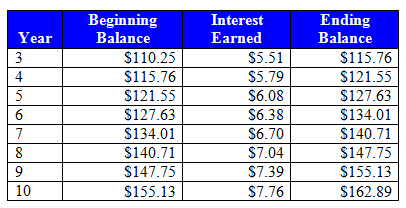At the end of 10 years, you would have \$162.89 in the account by doing absolutely nothing. But it gets better.

### If Interest Compounds Monthly

The example above assumes that the bank pays interest on the balance at the end of the year.  A bank usually pays interest on your account balance at the end of every month. The bank divides the annual interest rate (5%) by 12 months, then applies that rate to your balance at the end of each month.

In year one, this is what happens: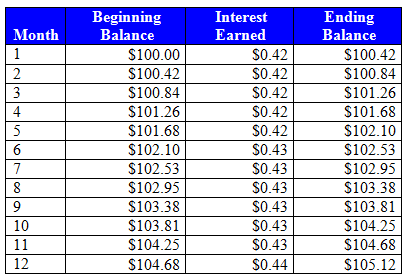Notice that when the bank compounded the balance annually, you only had \$105 at the end of year one. But if the bank compounds monthly, you have \$105.12 at the end of year one.

It may not sound like much, but consider the effect on a much larger balance of \$500,000.  At the end of 10 years, the investor would have \$814,447.13 (assuming the interest compounds annually). But, they’d have \$823,504.75 – a full \$9,057.62 more – if the interest compounded monthly.

## When High Frequency of Compounding Interest doesn’t Work for You

Compounding interest frequency doesn't just affect how much interest you can earn, it also affects how much interest you pay.

For example, if a \$1,000 savings account had been a \$1,000 loan, the amount of interest you pay would be influenced by the frequency that your bank compounded the rate. The important lesson here is that the more frequently compounding occurs, the more interest is earned (or paid) on a balance. Some credit cards even compound interest daily, which greatly affects the borrower's balance owed.

## What Is Continuous Compound Interest?

Continuous compound interest is the mathematical limit of the compound interest formula. The idea is that it’s compounded in every possible time increment instead of set periods.

Continuous compound interest is more theoretical than practical, as you will never see a bank or loan work this way.

## Simple Interest vs Compound Interest

Simple interest is always based on the principal balance (original deposit). Compound interest is based on the principal balance and the interest that’s added each time compounding occurs.

The formula for simple interest is: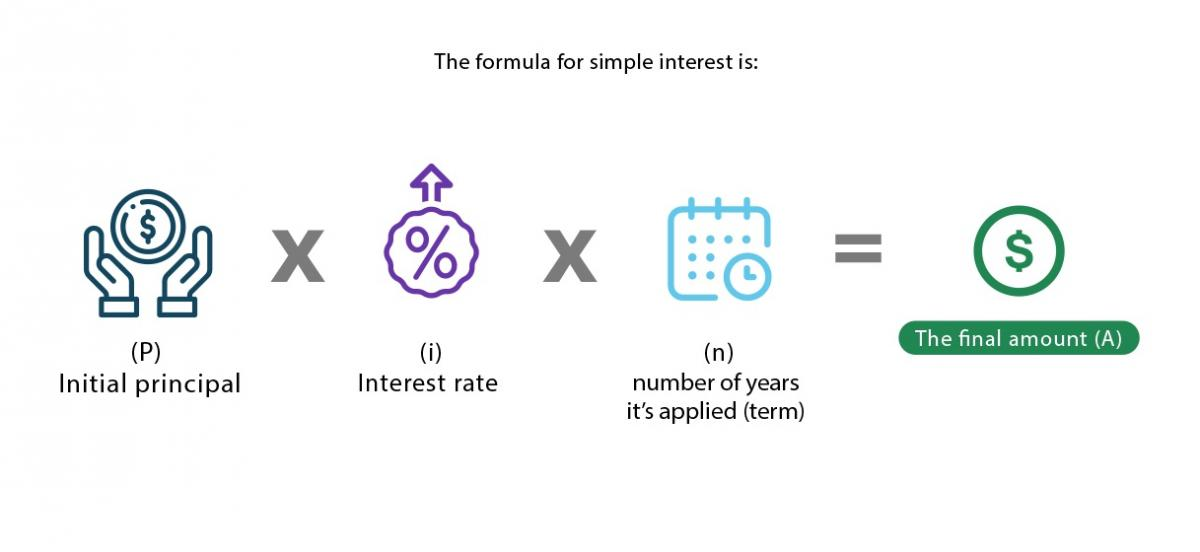Mortgages and auto loans are examples where simple interest is used instead of compounding interest. (That would make it difficult to ever pay off a home or car!)

## What to Look for in Compounding Interest

This definition only covered a few of the many interest calculation methods out there.  Borrowers should compare lender offers, and investors should compare investment offers by carefully reading disclosures. The Truth in Lending Act (TILA) is in place to assure that companies disclose all credit terms for borrowers.

Investors should understand how an institution’s choice of interest-calculation methods can affect the amount of interest applied to their account. For example, a Certificate of Deposit (CD) may have simple interest or compounding interest (compounding interest will build more wealth over time for the investor).

All of our content is verified for accuracy by Mark Herman, CFP and our team of certified financial experts. We pride ourselves on quality, research, and transparency, and we value your feedback. Below you'll find answers to some of the most common reader questions about Compound Interest.
##### Be the first to ask a question

Mark Herman, CFP - 145Mark Herman has been helping friends with financial questions since serving as an Army helicopter pilot. Since then, he’s gained valuable experience in the corporate world before moving on to become a Certified Financial Planner™.